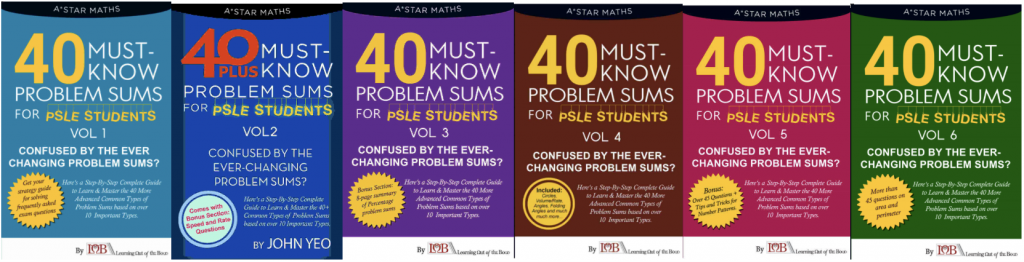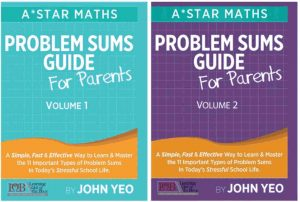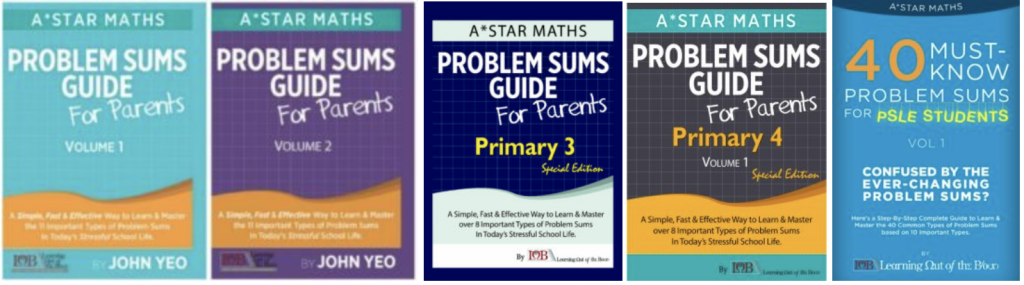# Your Child’s Guidebooks (by primary level)

Many parents had gotten our books and they had said it’s a turning point for them. So we want to make it easier for you to choose them – based on Primary level.

:

# P1/2

:

## Differences between 40 sums

#### Vol 1, 2, 3, 4, 5 and 6 (*suitable for P5/6)All cover different topics.

• Vol 1 covers 11 must-know level 1/ basic problem sums types.
• Vol 2 contains tips and tricks on the very challenging questions involving speed and algebra and it has the more challenging level 2 problem sums types.
• Vol 3 covers percentages problem sums too. It comes with an 8-page summary with the must-know smart tips on solving percentages problem sums fast.
• Vol 4 cover challenging problem sums on circles, volume/ rate, angles/ folding angles and much much more.
• Vol 5 covers number patterns too along with other tougher problem sums which P6s will eventually see in their exam papers.
:

## Differences between Problem Sums Guide – Vol 1 and 2

#### Vol 1 and 2 (*suitable for P3-6)• Volume 1 covers the 11 must-know level one problem sums types that all P5s must know by Term 1. (Students started to learn some of these concepts since P3 and all these concepts will be tested till your child is in P6.)
• Volume 2 covers the level 2 problem sums types that P5s will eventually see in their exam papers as they get tougher.
:

## Differences between Problem Sums Guide Vol 1 and 2 and the other Primary Level specific problem sums guidebooks.• Problem Sums Guide for Parents (Volume 1) covers the 11 must-know level one problem sums types that all P5s must know by Term 1. (Students started to learn some of these concepts since P3 and all these concepts will be tested till your child is in P6.)
• Problem Sums Guide for Parents (Volume 2) covers the level 2 problem sums types that P5s will eventually see in their exam papers as they get tougher.
• These two problem sums guidebooks provide your child with a complete understanding of the various important problem sums types and they give your child a stronger foundation in problem sums solving.

• The other 3 books (Problem Sums Guide for Parents (Primary 3), Problem Sums Guide for Parents (Primary 4) and 40 must-know problem sums for PSLE students) contain problem sums tested at that specific level.
• In short, parents get the Problem Sums Guide for Parents (Volume 1 and 2) to help their children have a stronger foundation in problem sums and they get the level specific guidebooks so that their children are well-prepared to tackle the tougher problem sums in their exam papers which are set according to the difficulty level of their primary level. (Use these and your child won’t be caught by surprise how TOUGH the problem sums are going to be in their actual exams. Yes, the problem sums are getting TOUGHER!)
:

## Difference between Primary Level specific problem sums guidebooks and the 51 sums##### Let’s use the Primary 4 Problem Sums Guide for Parents and 51 must-know problem sums as examples.
• Problem Sums Guide for Parents (Volume 1) covers the 17 types of problem sums with more advanced levels (your child should know all these by P4)
• The questions your child will see in his exam papers is endless. 51 must-know problem sums guidebook covers another 51 frequently asked questions in P4 exams.
• If you see 51 must-know problem sums guidebook for other primary levels, it serves the same purpose by providing your child with a summary book (like “ten years series”) for easy exam revision.
:

## Differences between Problem Sums Guide and 101 must know direction questions• Problem Sums Guide for Parents covers problem sums only. (you can see problem sums in both paper 1 and 2. The myth is problem sums are only tested in paper 2. So it’s not true. Problem sums can be found in any part of your child’s exam paper.)
• 101 must-know direction questions PSLE guide covers the direct/ 1 to 2-mark short structured questions. We have seen hundreds of exam papers and these are the frequently asked questions.
:

## Differences between Math Jungle

#### Vol 1 and 2 (*suitable for P1-3)• Volume 1 covers P1/2 problem sums like more/ less than and part-whole.
• Volume 2 covers P1/2/3 problem sums like more/ less than, part-whole and guess and check, before-after concept and other must-know P3 math concepts
: